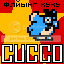-=+=- -=+=- -=+=- -=+=- -=+=- -=+=- -=+=- -=+=- -=+=- -=+=- -=+=- -=+=- -=+=- -=+=- -=+=- -=+=- -=+=- -=+=- -=+=- -=+=- -=+=- -=+=- -=+=- -=+=- -=+=- -=+=- -=+=- -=+=- -=+=- -=+=- (c) WidthPadding Industries 1987 0|698|0 -=+=- -=+=- -=+=- -=+=- -=+=- -=+=- -=+=- -=+=- -=+=- -=+=- -=+=- -=+=- -=+=- -=+=- -=+=- -=+=- -=+=- -=+=- -=+=- -=+=- -=+=- -=+=- -=+=- -=+=- -=+=- -=+=- -=+=- -=+=- -=+=- -=+=- Socoder -> Cobra -> Vertical bar effect Posted : Sunday, 20 April 2008, 17:28 Hotshotwhy it isnt working program uses pure2d,keyset VAR Screen:Integer a :integer b :integer c :integer ang1 :integer ang2 :integer Bar :element img :element BEGIN OpenScreen(640,480) bar = CreateImage( 16, 16 ) For a = 0 To 7 PenColor(ToRGBA(255-a*28,255-a*28,0), ScreenBuffer) Rect(7-a,0,1,16,ToRGBA(Rand(0),Rand(0),Rand(0),Rand(0)),True) Rect (8+a,0,1,16,ToRGBA(Rand(0),Rand(0),Rand(0),Rand(0)),True) Next img = CreateImage( 640, 480 ) While Not KeyDown(VK_SPACE) PenColor(ToRGBA(0,0,0), ScreenBuffer) Rect(0,464,640,16,ToRGBA(Rand(0),Rand(0),Rand(0),Rand(0)),True) b = ang1 c = ang2 PenColor(ToRGBA(255,0,0), ScreenBuffer) For a = 0 To 15 Drawimage (bar, 320+Sin( b ) * 200 + Sin( c ) * 100, 464) b = b + 8 c = c - 5 Next DrawImage(img, 0, 0) ang1 = ang1 + 1 If ang1 > 359 Then ang1 = ang1 - 360 ang2 = ang2 + 1 If ang2 > 359 Then ang2 = ang2 - 360 Flip Wend FreeImage(img) closescreen End --v Posted : Sunday, 20 April 2008, 18:01 flying_cuccoIts not showing *anything* because you are drawing everything in black. Not sure what it is *supposed* to look like. Lets look at the first bit bar = CreateImage( 16, 16 ) For a = 0 To 7 PenColor(ToRGBA(255-a*28,255-a*28,0), ScreenBuffer) Rect(7-a,0,1,16,ToRGBA(Rand(0),Rand(0),Rand(0),Rand(0)),True) Rect (8+a,0,1,16,ToRGBA(Rand(0),Rand(0),Rand(0),Rand(0)),True) Next --v Several times you pick this colour ToRGBA(Rand(0),Rand(0),Rand(0),Rand(0)) --v but Rand(0) is always 0, hence it is black. If you want any colour, try ToRGBA(Rand(255),Rand(255),Rand(255)) --v Rand(255) will choose anything from 0 to 255, which is the full range of colour component values. But I think maybe you want to get a gradient effect. Try taking out PenColor() command, as it doesn't work on Rect() -- rect specifies its own colour as an argument. Replace the random color in your Rect() commands with the colour from your PenColor() command ToRGBA(255-a*28,255-a*28,0) --v also, you want the Rect()s to draw on the bar image? So, add bar as the last argument, which specifies which image (or the screen) to draw to. So it looks like this bar = CreateImage( 16, 16 ) For a = 0 To 7 Rect(7-a,0,1,16,ToRGBA(255-a*28,255-a*28,0),TRUE,bar) Rect (8+a,0,1,16,ToRGBA(255-a*28,255-a*28,0),TRUE,bar) Next --v |edit| Oh, now there's a picture! Can you post/point to the original code? Posted : Sunday, 20 April 2008, 18:09 flying_cuccoIn cobra, you must set the target image in each command. I had to guess where the blitz SetBuffer ImageBuffer() commands were. Check the img and bar arguments added to the Rect() and DrawImage() commands. Colour is set in the command too. Check ToRGBA() arguments in the Rect() commands Finally I added a bit (commented below) to scroll the last line up, to make it look like the image you posted. I hope I guessed rightprogram uses pure2d,keyset VAR Screen:Integer a :integer b :integer c :integer ang1 :integer ang2 :integer Bar :element img :element BEGIN OpenScreen(640,480) bar = CreateImage( 16, 16 ) For a = 0 To 7 Rect(7-a,0,1,16,ToRGBA(255-a*28,255-a*28,0),TRUE,bar) Rect (8+a,0,1,16,ToRGBA(255-a*28,255-a*28,0),TRUE,bar) Next img = CreateImage( 640, 480 ) While Not KeyDown(VK_SPACE) //scroll image up DrawImage(img,0,-16,img) Rect(0,464,640,16,ToRGBA(0,0,0),TRUE,img) b = ang1 c = ang2 For a = 0 To 15 Drawimage (bar, 320+Sin( b ) * 200 + Sin( c ) * 100, 464,img) b = b + 8 c = c - 5 Next DrawImage(img, 0, 0) ang1 = ang1 + 1 If ang1 > 359 Then ang1 = ang1 - 360 ang2 = ang2 + 1 If ang2 > 359 Then ang2 = ang2 - 360 Flip Wend FreeImage(img) closescreen End --v Posted : Sunday, 20 April 2008, 19:01 HotshotwhoaExcellent flying_cucco thank you very muchCobra Version Screenshot